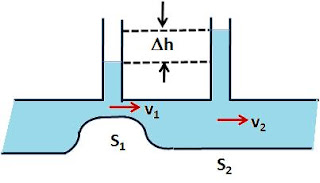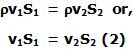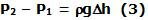## Sunday, May 3, 2009

### Irodov Problem 1.316Since the entire tube is at the same horizontal level, from Bernoulli's equation we have,The mass of water flowing per unit time through cross sections 1 and 2 must be exactly the same since there is no leakage. Hence, we have,The difference is pressures P2 - P1 is given be the difference in heights to which the water columns rise in the two cross-sections since both of them are exactly balanced by the same atmospheric pressure. Hence we have,From (1), (2) and (3) we obtain,The volume of water flowing per unit time is given by,